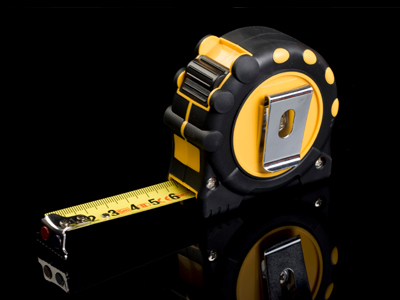There are 100cm in a metre and there are 1000m in a kilometre.

# Year 2 Fractions - Lengths

This quiz addresses the requirements of the National Curriculum KS1 Maths and Numeracy for children aged 6 and 7 in year 2. Specifically this quiz is aimed at the section dealing with finding fractions of lengths.

Understanding fractions of length means firstly being able to halve or quarter by dividing by 2 or 4. Applying this to a unit of measurement may mean having to convert units - for example, if asked to find half of 1m, it would first be sensible to recognise that 1m = 100cm.

Question 1
John walks 4 km to school. A 14 of the way to school is a sweet shop. How far is it from John’s home to the sweet shop?
1 km
2 km
4 km
8 km
To find a quarter of a number, divide it by 4 - or half it and half it again
Question 2
Jim ran 112 km. Ben ran 114 km. How much further did Jim run than Ben?
1 km
12 km
14 km
34 km
Two quarters are the same as a half
Question 3
What is 14 of a kilometre?
25cm
25m
250cm
250m
1 km is 1000m. Half of this is 500m, so a quarter is 250m
Question 4
I measure my pencil on Monday and it is 16cm long. By Friday, it is only half of this length. How long is my pencil on Friday?
10cm
8cm
4cm
12cm
Half of 16 is 8, so the pencil must be 8cm long
Question 5
May grows her sunflower to 80cm. Jake's is only 14 of this height. How tall is Jake's sunflower?
80cm
60cm
40cm
20cm
To find a quarter, divide by 4
Question 6
Cubes measure 3cm each. I make a row of 10 cubes. Then I break the row in half. How long is each half of the row?
10cm
12cm
15cm
13cm
3cm x 10 = 30cm. Half of 30 is 15
Question 7
A roll of tape measures 12m. I use 34 of the tape. How much is left?
5 m
3 m
2 m
6 m
To find a quarter, divide 12 by 4 to get an answer of 3. If 14 is 3, then 34 is 9, leaving 3m of tape
Question 8
I have a piece of ribbon which is 64cm long. I cut it in half - how long will each piece be?
40cm
23cm
28cm
32cm
Half of 64 is 32
Question 9
Sam walks 1 km to school every day. She meets her best friend exactly halfway. How far does Sam walk before she meets her friend?
250m
500m
2km
100km
1 km is 1000m. Half of 1000 is 500
Question 10
Harry is 120 cm tall. His little brother is half this height. How tall is Harry’s brother?
40cm
60cm
80cm
240cm
Half of 120 is 60